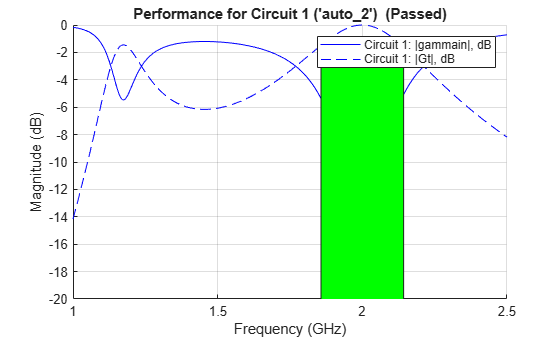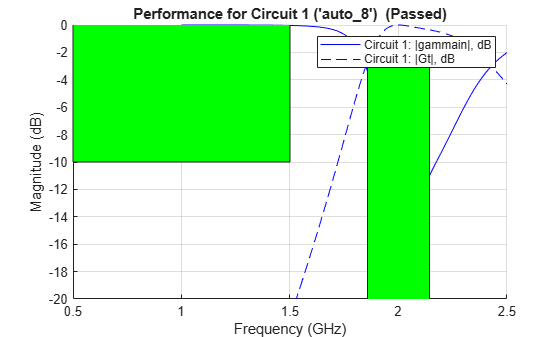# rfplot

Plot input reflection coefficient and transducer gain of matching network

Since R2019a

## Syntax

``rfplot(mnobj)``
``rfplot(mnobj,frequencylist)``
``rfplot(mnobj,frequencylist,circuitindices)``
``hline = rfplot(mnobj,frequencylist,circuitindices)``

## Description

````rfplot(mnobj)` plots the input reflection coefficient and transducer gain of the matching network when cascaded between source and load impedances. This plot compares the actual performance of the network against the performance goals.```
````rfplot(mnobj,frequencylist)` plots using values calculated at each frequency in the `frequencylist`.```

example

````rfplot(mnobj,frequencylist,circuitindices)` plots performances of matching networks specified in the `circuitindices`.```
````hline = rfplot(mnobj,frequencylist,circuitindices)` returns a cell array of line handle vectors, one cell for each circuit plotted.```

## Examples

collapse all

Create a dipole antenna and create the S-parameters of the antenna. This example requires Antenna Toolbox.

```d = dipole('Length', 0.103, 'Width',0.0022); freq = linspace(0.5e9,2.5e9,1001); sd = sparameters(d, freq);```

Alternatively, load S-Parameters from the MAT file

`% load('sparams_dipole.mat')`

Create a matching network from the S-parameters.

```n = matchingnetwork('LoadImpedance',sd,'Components',3,... 'LoadedQ',7,'CenterFrequency',2e9);```

Get the evaluation parameters of the network.

`t = getEvaluationParameters(n)`
```t=1×6 table Parameter Comparison Goal Band Weight Source _________ __________ ______ _________________________ ______ _____________ {'Gt'} {'>'} {[-3]} {[1.8571e+09 2.1429e+09]} {} {'Automatic'} ```

Plot the reflection coefficient and transducer gain of the matching network circuit 1 , at a frequency range of 1 GHz to 2.5 GHz.

`rfplot(n, (1e9:0.001e9:2.5e9),1);`Add a new evaluation parameter to compare the transducer gain to have a cut-off of less than -10 dB. Use a frequency range of 0.5 GHz to 1.5 GHz. Plot the comparisons.

```n = addEvaluationParameter(n, 'Gt', '<', -10, [0.5e9 1.5e9], 1); t = getEvaluationParameters(n)```
```t=2×6 table Parameter Comparison Goal Band Weight Source _________ __________ _______ _________________________ ______ __________________ {'Gt'} {'>'} {[ -3]} {[1.8571e+09 2.1429e+09]} {} {'Automatic' } {'Gt'} {'<'} {[-10]} {[ 500000000 1.5000e+09]} {} {'User-specified'} ```
`rfplot(n, (1e9:0.001e9:2.5e9),1);`Clear evaluation parameters.

```n = clearEvaluationParameter(n,1); t = getEvaluationParameters(n)```
```t=1×6 table Parameter Comparison Goal Band Weight Source _________ __________ _______ ________________________ ______ __________________ {'Gt'} {'<'} {[-10]} {[500000000 1.5000e+09]} {} {'User-specified'} ```

## Input Arguments

collapse all

Matching network, specified as a `matchingnetwork` object.

Data Types: `char` | `string`

Frequency values at which to plot the performance of the matching network, specified as a vector with each element in Hz.

Data Types: `double`

Index of the matching network circuit, specified as a vector or scalar.

Data Types: `double`

## Output Arguments

collapse all

Line handle of circuit plotted, returned as a cell array with one cell for each circuit plotted.

## Version History

Introduced in R2019a